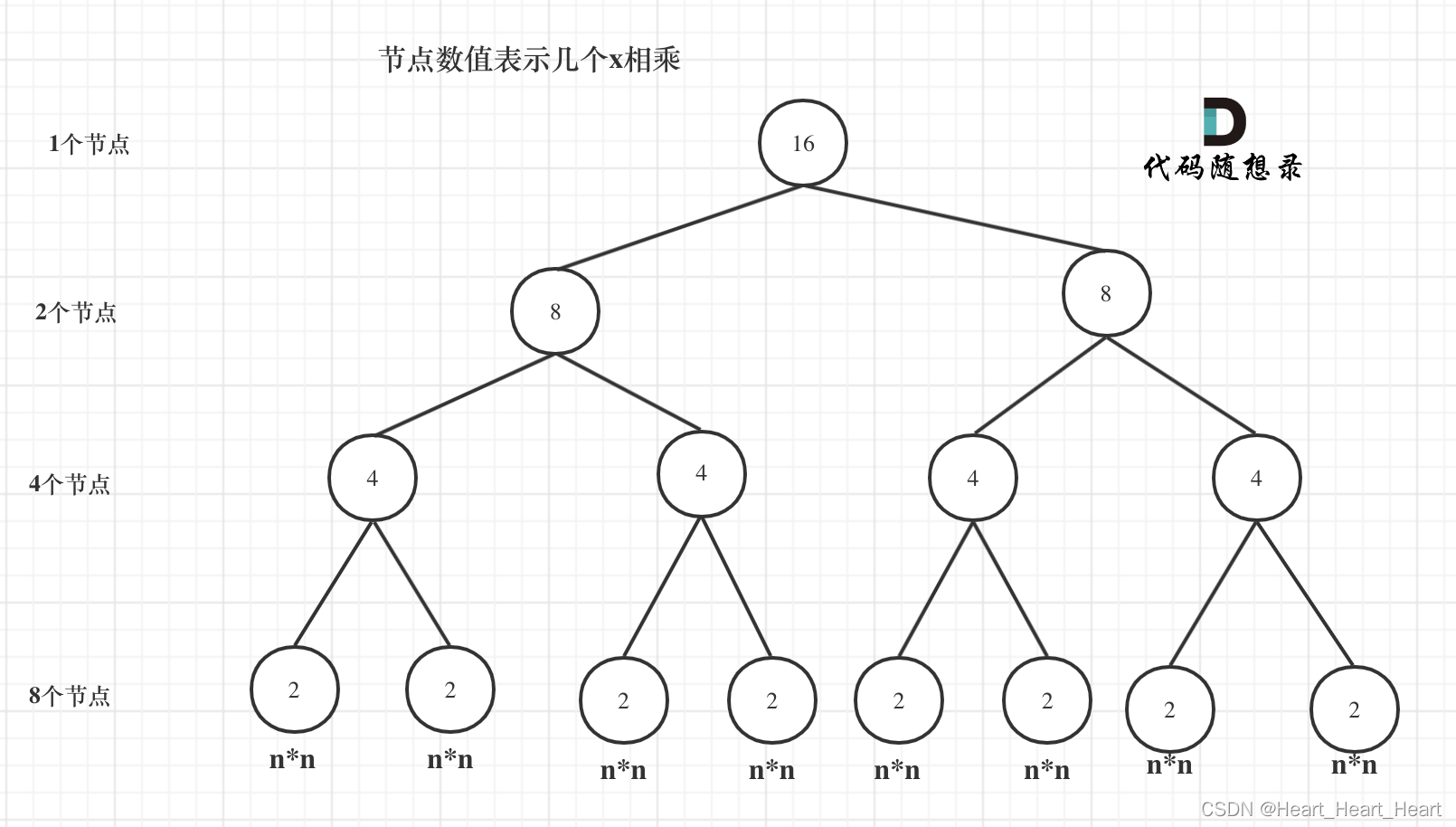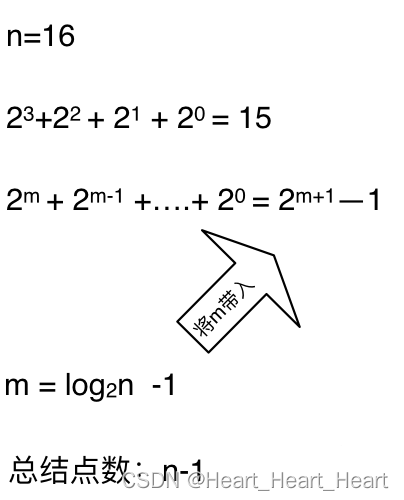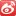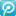• 2016-09-23 00:55:25

递归函数时间复杂度分析

(1) 递归执行过程
例子：求N!。
这是一个简单的”累乘”问题，用递归算法也能解决。
n! = n * (n - 1)! n > 1
0! = 1, 1! = 1 n = 0,1
因此，递归算法如下：

Java代码
fact(int n) {
if(n == 0 || n == 1)
return 1;
else
return n * fact(n - 1);
}
以n=3为例，看运行过程如下：
fact(3) —– fact(2) —– fact(1) —— fact(2) —–fact(3)
——————————> ——————————>
递归 回溯
递归算法在运行中不断调用自身降低规模的过程，当规模降为1，即递归到fact(1)时，满足停止条件停止递归，开始回溯(返回调用算法)并计算，从fact(1)=1计算返回到fact(2);计算2*fact(1)=2返回到fact(3)；计算3*fact(2)=6，结束递归。
算法的起始模块也是终止模块。
(2) 递归实现机制
每一次递归调用，都用一个特殊的数据结构”栈”记录当前算法的执行状态，特别地设置地址栈，用来记录当前算法的执行位置，以备回溯时正常返回。递归模块的形式参数是普通变量，每次递归调用得到的值都是不同的，他们也是由”栈”来存储。
(3) 递归调用的几种形式
一般递归调用有以下几种形式(其中a1、a2、b1、b2、k1、k2为常数)。
<1> 直接简单递归调用： f(n) {…a1 * f((n - k1) / b1); …};

<2> 直接复杂递归调用： f(n) {…a1 * f((n - k1) / b1); a2 * f((n - k2) / b2); …};
<3> 间接递归调用： f(n) {…a1 * f((n - k1) / b1); …}，
g(n) {…a2 * f((n - k2) / b2); …}。
2. 递归算法效率分析方法
递归算法的分析方法比较多，最常用的便是迭代法。
迭代法的基本步骤是先将递归算法简化为对应的递归方程，然后通过反复迭代，将递归方程的右端变换成一个级数，最后求级数的和，再估计和的渐进阶。
<1> 例：n!
算法的递归方程为： T(n) = T(n - 1) + O(1);
迭代展开： T(n) = T(n - 1) + O(1)
= T(n - 2) + O(1) + O(1)
= T(n - 3) + O(1) + O(1) + O(1)
= ……
= O(1) + … + O(1) + O(1) + O(1)
= n * O(1)
= O(n)
这个例子的时间复杂性是线性的。
<2> 例：如下递归方程：

  T(n) = 2T(n/2) + 2, 且假设n=2的k次方。
T(n) = 2T(n/2) + 2
= 2(2T(n/2*2) + 2) + 2
= 4T(n/2*2) + 4 + 2
= 4(2T(n/2*2*2) + 2) + 4 + 2
= 2*2*2T(n/2*2*2) + 8 + 4 + 2
= ...
= 2的(k-1)次方 * T(n/2的(i-1)次方) + \$(i:1~(k-1))2的i次方
= 2的(k-1)次方 + (2的k次方)  - 2
= (3/2) * (2的k次方) - 2
= (3/2) * n - 2
= O(n)
这个例子的时间复杂性也是线性的。


<3> 例：如下递归方程：

  T(n) = 2T(n/2) + O(n), 且假设n=2的k次方。
T(n) = 2T(n/2) + O(n)
= 2T(n/4) + 2O(n/2) + O(n)
= ...
= O(n) + O(n) + ... + O(n) + O(n) + O(n)
= k * O(n)
= O(k*n)
= O(nlog2n) //以2为底

一般地，当递归方程为T(n) = aT(n/c) + O(n), T(n)的解为：
O(n)          (a<c && c>1)
O(nlog2n)     (a=c && c>1) //以2为底
O(nlogca)     (a>c && c>1) //n的(logca)次方，以c为底


上面介绍的3种递归调用形式，比较常用的是第一种情况，第二种形式也有时出现，而第三种形式(间接递归调用)使用的较少，且算法分析
比较复杂。 下面举个第二种形式的递归调用例子。
<4> 递归方程为：T(n) = T(n/3) + T(2n/3) + n
为了更好的理解，先画出递归过程相应的递归树：
n ——–> n
n/3 2n/3 ——–> n
n/9 2n/9 2n/9 4n/9 ——–> n
…… …… …… ……. ……
——–
总共O(nlogn)
累计递归树各层的非递归项的值，每一层和都等于n，从根到叶的最长路径是：

  n --> (2/3)n --> (4/9)n --> (12/27)n --> ... --> 1
设最长路径为k，则应该有：

(2/3)的k次方 * n = 1
得到 k = log(2/3)n  // 以(2/3)为底
于是 T(n) <= (K + 1) * n = n (log(2/3)n + 1)
即 T(n) = O(nlogn)
由此例子表明，对于第二种递归形式调用，借助于递归树，用迭代法进行算法分析是简单易行的。

更多相关内容
• 时间复杂度计算 此笔记来源于左神算法，只用做笔记使用，已注明来源。 此事件复杂度只适用于递归算法，并且递归过程中要求数据规模大体相同 T(N)=a∗T(Nb)+O(Nd)T(N)=a*T\left(\frac{N}{b}\right)+O(N^d)T(N)=a∗T...

## 时间复杂度计算

此笔记来源于左神算法，只用做笔记使用，已注明来源。

此事件复杂度只适用于递归算法，并且递归过程中要求数据规模大体相同

T ( N ) = a ∗ T ( N b ) + O ( N d ) T(N)=a*T\left(\frac{N}{b}\right)+O(N^d)

• 1 当 log ⁡ b a > d \log_ba>d 复杂度为 O ( N log ⁡ b a ) O\left(N^{\log_ba}\right)
• 2 当 log ⁡ b a < d \log_ba<d 复杂度为 O ( N d ) O\left(N^d\right)
• 3 当 log ⁡ b a = d \log_ba=d 复杂度为 O ( N d × log ⁡ N ) O\left(N^d\times{\log{N}}\right)

a为将数据分成几块，b为每块数据占用总数据的数据量， O ( N d ) O(N^d) 为每迭代的时间复杂度,然后计算d的值

展开全文算法
• 今天学习的是递归函数时间复杂度的简单学习 从求x的n次方这道题的角度了解时间复杂度递归函数 1.首先是求x的n次方的最一般解法 （for循环解法) 这种解法最容易想到 循环了n次 每次仅有一次运算 它的时间复杂度...

今天学习的是递归函数时间复杂度的简单学习

## 从求x的n次方这道题的角度了解时间复杂度和递归函数

### 1.首先是求x的n次方的最一般解法（for循环解法)

这种解法最容易想到

循环了n次 每次仅有一次运算 它的时间复杂度O(n)

// 使用循环 时间复杂度
public static int function0(int x, int n){
int result = 1;
for (int i = 1; i <= n; i ++){
result *= x;
}
return result;
}

### 2.然后是求x的n次方的递归解法（递归解法)

使用递归法实现，它的时间复杂度又是多少呢？

该递归方法中 递归的次数 为n次 每次递归的操作1次

根据递归算法的时间复杂度 = 递归的次数 * 每次递归中的操作次数

可得它的时间复杂度也是O(1)

/**
* 递归写法
* 递归算法的时间复杂度 = 递归的次数 * 每次递归中的操作次数
* 时间复杂度   o(n)         n             1
*/
public static int function1(int x, int n){
if (n <= 0){
return 0;
}
if (n == 1){
return x;
}
return function1(x, n-1) * x;
}

总结：这次递归并没有使得算法的时间复杂度降低

3.那么换一种递归方法（第二种递归解法)

使用第二种递归解法

运用 二分法 将 n 次方 变成 两个 n / 2 次相乘


/**
* 优化递归算法
* 运用 二分法 将 n 次方 变成 两个 n / 2 次相乘 进行优化
*
* 时间复杂度依旧是    o(n)
*/
public static int function2(int x, int n){
if (n == 0){
return 0;
}
if (n == 1){
return x;
}
if (n % 2 == 1){
return function2(x, n / 2) * function2(x, n / 2) * n;
}
return function2(x, n / 2) * function2(x, n / 2);
}

这种方法的时间复杂度仍为O(n)

### 具体看《代码随想录》中作者的解释

我们来分析一下，首先看递归了多少次呢，可以把递归抽象出一棵满二叉树。

可以用一棵满二叉树来表示（为了方便表示，选择n为偶数16）。当前这棵二叉树就是求x的n次方，n为16的情况，n为16的时候，进行了多少次乘法运算呢？

这棵树上每一个节点就代表着一次递归并进行了一次相乘操作，所以进行了多少次递归的话，就是看这棵树上有多少个节点。

熟悉二叉树话应该知道如何求满二叉树节点数量，这棵满二叉树的节点数量就是2^3 + 2^2 + 2^1 + 2^0 = 15，可以发现：**这其实是等比数列的求和公式，这个结论在二叉树相关的面试题里也经常出现**。

这么如果是求x的n次方，这个递归树有多少个节点呢，如下图所示：(m为深度，从0开始)时间复杂度忽略掉常数项-1之后，这个递归算法的时间复杂度依然是O(n)

### 4.优化上一个递归方法（第二种递归解法优化)

仅仅将function3(x, n / 2)抽取出来，减少递归中操作的次数

就可以真正意义上的降低时间复杂度

该方法的时间复杂度就是O(logn)

/**
* 再优化递归函数
* 每次递归仅有一个递归调用
* 递归算法的时间复杂度 = 递归的次数(也就是二叉树的深度) * 每次递归中的操作次数
*    o(logn)            logn                           1
*
*/
public static int function3(int x, int n){
if (n == 0){
return 0;
}
if (n == 1){
return x;
}
int t = function3(x, n / 2);
if (n % 2 == 1){
return t * t * n;
}
return t * t;
}

根据以上不断优化的四种不同情况，同一程序的时间复杂度只在最后一次才达到理想中的O(logn),这也给了我们优化算法的集中不同思路与启示，并不仅仅是优化了方法就一定会降低代码的时间复杂度，更多的还需要考虑程序运行的真实情况等等

展开全文算法 leetcode 数据结构
• 这个方法为估计形如：T(n) = aT(n/b) + f(n)其中，a≥1和b≥1，均为常数，f(n)是一个确定的正函数。在f(n)的三类情况下，我们有T(n)的渐近估计式：1.若对于某常数ε>0，有f(n) = O(nlogb a-ε )，则T(n) = O...

这个方法为估计形如：

T(n) = aT(n/b) + f(n)

其中，a≥1和b≥1，均为常数，f(n)是一个确定的正函数。在f(n)的三类情况下，我们有T(n)的渐近估计式：

1.若对于某常数ε>0，有f(n) = O(nlogb a-ε )，则T(n) = O(nlogb a )

2.若f(n) = O(nlogb a )，则T(n) = O(nlogb a *logn)

3.若f(n) = O(nlogb a+ε )，且对于某常数c>1和所有充分大的正整数n，有af(n/b)≤cf(n)，则T(n)=O(f(n))。

设T(n) = 4T(n/2) + n，则a = 4，b = 2，f(n) = n，计算得出nlogb a = nlog2 4 = n2 ，而f(n) = n = O(n2-ε )，此时ε= 1，根据第1种情况，我们得到T(n) = O(n2 )。

这里涉及的三类情况，都是拿f(n)与nlogb a 作比较，而递归方程解的渐近阶由这两个函数中的较大者决定。在第一类情况下，函数nlogb a 较大，则T(n)=O(nlogb a )；在第三类情况下，函数f(n)较大，则T(n)=O(f (n))；在第二类情况下，两个函数一样大，则T(n)=O(nlogb a *logn)，即以n的对数作为因子乘上f(n)与T(n)的同阶。

分享到：2011-02-21 14:03

浏览 2260

评论

展开全文• ## 彻底搞懂递归的时间复杂度

千次阅读 多人点赞 2021-09-15 00:22:41
笔者编码10载，面过很多程序员简历上写着熟悉数据结构和算法，但是对于时间复杂度稍微深入点的问题，都回答的不怎么样，其实还是没懂 搞懂算法时间复杂度是一个优先程序员的分水岭 先来看letcode一道题， 泰波那契...算法 数据结构
• 任何一个分治或者递归函数都可以算出它的时间复杂度 关键4种 二分查找:发生在一个数列本身有序的时候,要在有序的数列中找到你要的目标数,所以每次一分为2只查一边,最后时间复杂度是O(logN) 从 T(n) = T(n/2) + O(1...
• ## 递归算法时间复杂度分析

万次阅读 多人点赞 2018-09-17 16:16:59
递归算法时间复杂度分析 时间复杂度： 一般情况下，算法中基本操作重复的次数就是问题规模n的某个函数f（n），进而分析f（n）随n的变化情况并确定T（n）的数量级。这里用‘o’来表示数量级，给出算法时间复杂度。 T...
• Python递归-时间复杂度 递归也是常见算法之一 其时间复杂度一般认为O(logn)，但递归算法的时间复杂度本质上是要看: 递归的次数 * 每次递归中的操作次数 举例面试题：求x的n次方 思路一：for循环 def x_n(x,n): """ ...python 排序算法 leetcode
• （1）计算x的n次方的幂运算 ...double pow( double x, int n )...指数级的算法，时间复杂度O（2^n） （3）递归中多次递归调用
• T(N) = a * T(N/b) + O(N^d)(其中的a、b、d都是常数)的递归函数，可以直接通过master公式来确定时间复杂度 ①当d<logb a时，时间复杂度为O(n^(logb a)) ②当d=logb a时，时间复杂度为O((n^d)*logn) ③当d>...算法
• 第一步走了一个台阶或第一步走了两个台阶，到下一个台阶也是类似，故这是一个递归。 n个台阶就是，走了一个台阶后加剩下n-1台阶的走法，走了两个台阶后剩下n-2台阶的走法， f(n)=f(n-1)+f(n-2) 终止条件：只剩一...
• 引言：时间复杂度的求解，在此都是以实例进行讲解，各位读者可以从中慢慢理解；以下所有的案例都是以Python语言编写！案例一：求a的n次方代码如下：def exp1(a,n):if n == 1:return aelse:return a*exp2(a,n-1)分析...
• 题目：求x的n次方 解法一：for循环 function fun1(x,n){ ...此时时间复杂度为O(n) 解法二：递归 function fun2(x,n){ if(n === 0) return 1; // 先求出 x的n/2 次方 let t = fun2(x,n/2); if(n%2){ retu排序算法 动态规划 算法
• 下面介绍几种递归函数中的算法时间复杂度分析的方法 0.递推法 这种方法是最简单的，也是最容易理解的一种方法，对于一个递归函数，我们可以利用他的递归方程，将其递推到常量的情况下，这样子就很容易求解他的时间...算法 二叉树 数据结构
• ## 公式法求递归算法的时间复杂度

千次阅读 多人点赞 2020-03-24 09:25:24
公式法可以说是计算递归函数复杂度最方便的工具，当递归函数的时间执行函数满足如下的关系式时，我们可以利用公式法： T(n)=a⋅T(nb)+f(n)T(n)=a \cdot T\left(\frac{n}{b}\right)+f(n)T(n)=a⋅T(bn​)+f(n) 其中，f...数据结构 算法 python
• 递归函数时间复杂度分析(1) 递归执行过程(2) 递归实现机制(3) 递归调用的几种形式2. 递归算法效率分析方法 1.递归函数时间复杂度分析 (1) 递归执行过程 例子：求N!。 这是一个简单的"累乘"问题，用递归算法也能解决...java
•Master公式
• 1.递归算法求时间复杂度步骤 ...如果在满足递归条件这一类中，计算执行次数的时候，发现要调用递归函数的话，就直接用函数记这一次的执行次数如+T（n+1) ③根据题意，将变量从0开始的时候执行，一直执行到 ...数据结构
• 例题：用时间复杂度为O(log^n)的算法求解x的n次方 1.常见递归解法： def function1(x, n): if n==0: return 1 else: return function1(x, n-1)*x 该方法的时间复杂度为O(n) 2.区分n的奇偶性 def function2(x,...算法 数据结构
• 函数递归调用的时间复杂度 首先关于这个话题，我们先介绍一下递归时间复杂度两个概念： 递归 就我个人而言，我理解的概念就是在解决许多问题需要使用到许多重复的步骤的时候，就可以通过程序自身调用自身的方法...C语言
• 斐波那契数列——递归时间复杂度计算 画图：以F（6）为例：斐波那契数列到第六个数停止 每个节点运行都会开辟空间，使用时间 为了方便计算，把第五层的f（2）和f（1）放在第四层最右边，不会影响时间复杂度计算 ...
• ## 用递归树求解递归算法时间复杂度

万次阅读 多人点赞 2019-07-01 23:27:38
递归代码复杂度分析起来比较麻烦。一般来说有两种分析方法：递推公式和递归树。 1 递推公式法 归并排序的递推公式是： merge_sort(p…r) = merge(merge_sort(p…q), merge_sort(q+1…r)) 终止条件： p >= r ...
• 我们把复杂度理解成基本操作的执行次数，例如赋值是一次操作之类的。复杂度本身的大OO记号，就是一种渐进的表达，属于大概的定性分析，因此执行次数一般只考虑循环迭代所带来的基本操作执行次数。例如:void f() { ...
• 使用递归需要满足的条件 1、大问题可以分解成多个规模较小的子问题。 2、这些子问题的求解思路与原问题一致。 3、子问题的分解不能无限循环下去，存在终止条件。 为此写递归代码的思路为：根据问题的分解过程，找到...算法
• 0.复杂度度量 Mathematics is more in need of good notations than of new theorems. 与新定理相比，数学更需要好的符号。...执行时间随规模增长的变化趋势可以表示为输入规模的一个函数，称为该算法的时间...数据结构
• 这一段时间，我研究了一下算法的时间复杂度分析，感觉其中的递归分析挺有意思，就总结一下记录下来，以备以后随时复习查看。下面假设递归方程式已经给出了，仅仅说明如何计算递归方程的时间复杂度...